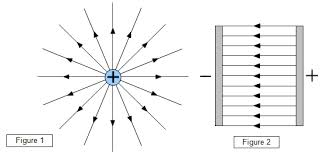# Edexcel Physics A2 Unit 4 Electric and Magnetic Fields

A set of succinct notes on Electric and Magnetic Fields. Used the CGP revision guide and my own notes to compile.

?

## Coloumb's Law

Charged objects have an ELECTRIC FIELD around them - this is the region where they can attract or reel other charges.

• Charge is measured in Coloumbs (C).
• Objects placed in electric fields experience a force.

COULOMBS LAW: F = kQ1Q2/r²     where    k = 1 / 4πε

where ε = permittivity between charges. For free space, ε = 8.85 x 10^-12  C²N^-1N^-2

This is an inverse square law - the further apart the charges are, the weaker the forces between them.

Also, the force on Q1 is equal and opposite to the force on Q21 of 3

## Radial and Uniform Electric FieldsFigure 1 - RADIAL FIELD                  Figure 2 - UNIFORM FIELD

Electric Field Strength is force per unit charge     E = F/Q

In a radial field E is inversely proportional to r² - to calculate the filed strength in a radial field combine Coloumb's Law and the above equation to get:    E=kQ/r²

In a uniform field E is the same everywhere   (E is uniform)   E = V/d

2 of 3

## Capacitors

CAPACITANCE is the amount of charge stored per volt    C = Q/V    and is measured in farads

Capacitors store energyIf the switch is flicked to A, the capacitor is charging - charges are deposited on one plate and the opposite charge is removed from the other plate. When switched to B, the capacitor discharges. You can find the energy stored in a capacitor by plotting a graph of V against Q and finding the area under the graph. This gives W = 1/2 QV.

Substitute Q=CV and you get: Q = 1/2CV²       s4bst5t4te V=Q/C and you get W = Q²/2C

3 of 3